Checkout JEE MAINS 2022 Question Paper Analysis : Checkout JEE MAINS 2022 Question Paper Analysis :

# Three Dimensional Shapes

In geometry, three-dimensional shapes or 3D shapes are solids that have three dimensions such as length, width and height. Whereas 2d shapes have only two dimensions, i.e. length and width. Examples of three-dimensional objects can be seen in our daily life such as cone-shaped ice cream, cubical box, a ball, etc. Students will come across different 3D shapes models in Maths.

Geometry is one of the practical sections of Mathematics that involves various shapes and sizes of different figures and their properties. Geometry can be divided into two types: plane and solid geometry. Plane geometry deals with flat shapes like lines, curves, polygons, etc., that can be drawn on a piece of paper. On the other hand, solid geometry involves objects of three-dimensional shapes such as cylinders, cubes, spheres, etc. In this article, we are going to learn different 3D shapes models in Maths such as cube, cuboid, cylinder, sphere and so on along with its definitions, properties, formulas and examples in detail.

## What are Three-Dimensional Shapes?

Shapes that can be measured in 3 directions are called three-dimensional shapes. These shapes are also called solids. Length, width, and height (or depth or thickness) are the three measurements of three-dimensional shapes. These are the part of three-dimensional geometry. They are different from 2D shapes because they have thickness. Several examples can be found in everyday life. Some of them are: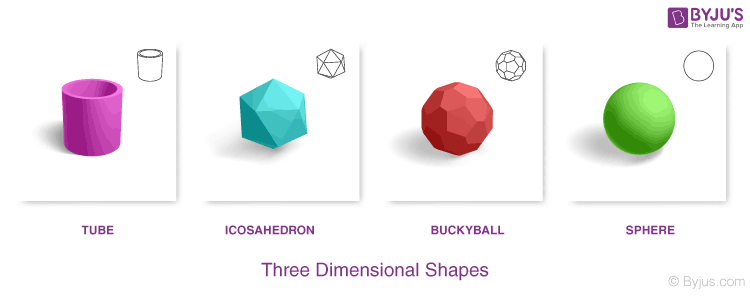### Solid Shapes in Maths

In Mathematics, the three-dimensional objects having depth, width and height are called solid shapes. Let us consider a few shapes to learn about them. You can find many examples of solid shapes around you, such as a mobile, notebook or almost everything you can see around is a solid shape.

## Faces, Edges, and Vertices of Three Dimensional Shapes

Three-dimensional shapes have many attributes, such as vertices, faces, and edges. The flat surfaces of the 3D shapes are called faces. The line segment where two faces meet is called an edge. A vertex is a point where three edges meet.

Also, read: Vertices, Edges and Faces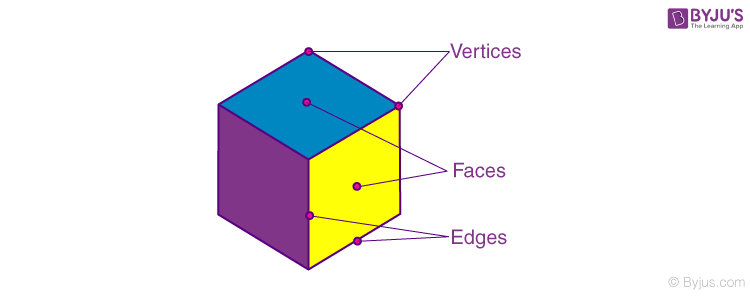Faces, Edges and Vertices

## List of Three-dimensional Shapes

The list of three-dimensional shapes are as follows:

 Three-dimensional Shapes Names: Cube Cuboid Cone Cylinder Sphere Pyramid Prism

Here, we are going to discuss the list of different three-dimensional shapes with their properties and the formulas of different 3D shapes.

### Cube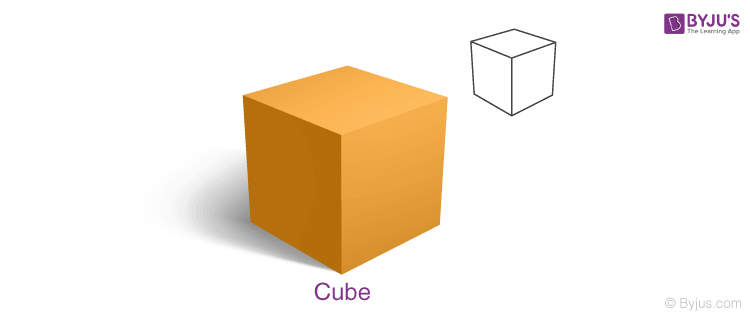A cube is a solid or three-dimensional shape which has 6 square faces. The cube has the following properties.

• All edges are equal
• 8 vertices
• 12 edges
• 6 faces

### Cuboid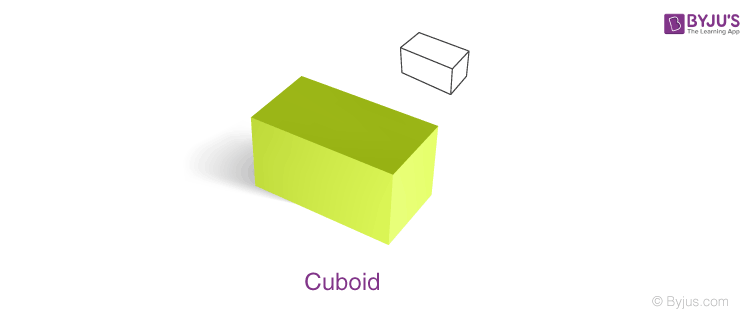A cuboid is also called a rectangular prism, where the faces of the cuboid are a rectangle in shape. All the angles measure 90 degrees. The cuboid has

• 8 vertices
• 12 edges
• 6 faces

### Prism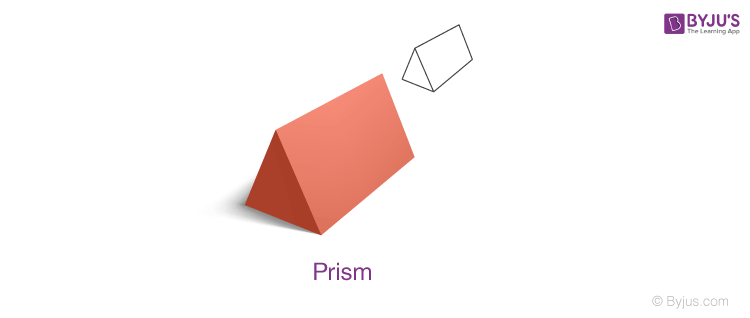A prism is a 3D shape which consists of two equal ends, flat surfaces or faces, and also has identical cross-section across its length. Since the cross-section looks like a triangle, the prism is generally called a triangular prism. The prism does not have any curve. Also, a prism has

• 6 vertices
• 9 edges
• 5 faces – 2 triangles and 3 rectangles

### Pyramid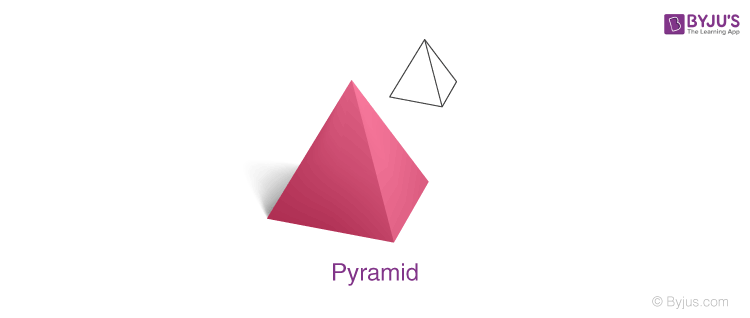A pyramid a solid shape, whose outer faces are triangular and meet to a single point on the top. The pyramid base can be of any shape such as triangular, square, quadrilateral or in the shape of any polygon. The most commonly used type of a pyramid is the square pyramid, i.e., it has a square base and four triangular faces. Consider a square pyramid, it has

• 5 vertices
• 8 edges
• 5 faces

### Cylinder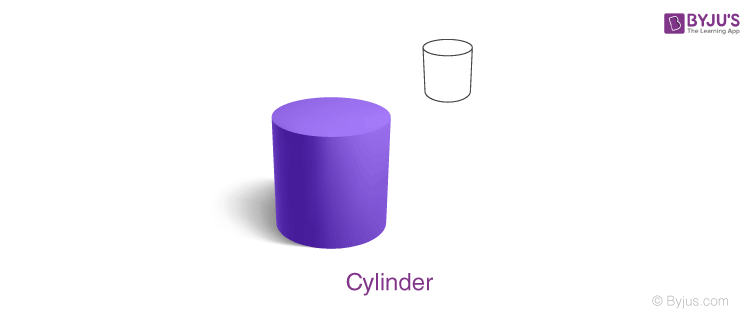A cylinder is defined as a three-dimensional geometrical figure which has two circular bases connected by a curved surface. A cylinder has

• No vertex
• 2 edges
• 2 flat faces – circles
• 1 curved face

### Cone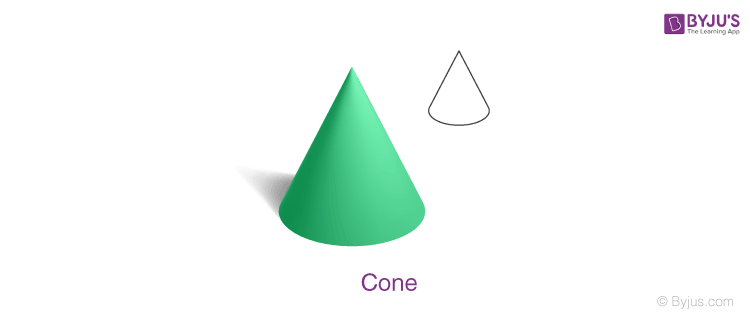A cone is a three-dimensional object or solid, which has a circular base and has a single vertex. The cone is a geometrical figure that decreases smoothly from the circular flat base to the top point called the apex. A cone has

• 1 vertex
• 1 edge
• 1 flat face – circle
• 1 curved face

### Sphere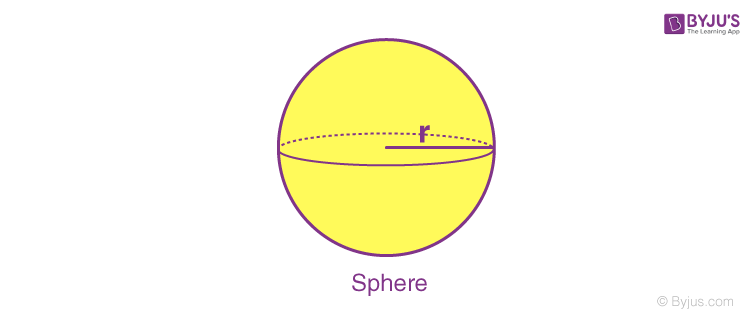A sphere is a three-dimensional solid figure which is perfectly round in shapes and every point on its surface is equidistant from the point is called the center. The fixed distance from the center of the sphere is called a radius of the sphere. A sphere has

• No vertex
• No edges
• 1 curved face

### 3D Shapes Model in Maths Project

If you know what three-dimensional shapes are, it would be easy for you to build a 3d shapes models in Maths such as projects for constructing a house or a building. This would be easy for the students to make as they can measure the rooms easily. Rest all they need is cardboard, glue, scissors and art supplies to make it look exactly like a mini house or building.

## Surface Area and Volume of 3D shapes

The two distinct measures used for defining the 3D shapes are:

• Surface Area
• Volume

Surface Area is defined as the total area of the surface of the three-dimensional object.  It is denoted as “SA”. The surface area is measured in terms of square units. The three different classifications of surface area are defined below. They are:

• Curved Surface Area (CSA) is the area of all the curved regions
• Lateral Surface Area (LSA) is the area of all the curved regions and all the flat surfaces excluding base areas
• Total Surface Area (TSA) is the area of all the surfaces including the base of a 3D object

Volume is defined as the total space occupied by the three-dimensional shape or solid object. The volume is denoted as “V”. It is measured in terms of cubic units.

 Shapes Surface Area Volume Cube The Surface Area of a Cube = 6a2 square units The volume of a Cube = a3  cubic units Cuboid The Surface Area of a Cuboid = 2(lb+bh+lh) Square units The volume of a Cuboid = lbh Cubic units Cylinder The Surface Area of a Cylinder = 2πr(h +r) Square units The curved surface area of a cylinder = 2πrh The volume of a Cylinder =  πr2 h Cubic units Cone Surface Area of a Cone = πr(r +√(r2+h2) Square units Curved surface area of a cone =πrl square units Slant height of a cone = l = √(r2+h2) units Volume of a Cone = ⅓ πr2h Cubic units Cylinder Surface Area of a Cylinder = 2πr(h +r) Square units Curved surface area of a cylinder = 2πrh Volume of a Cylinder =  πr2 h Cubic units Sphere Curved Surface Area of a Sphere = 2πr² Square units Total Surface Area of a Sphere = 4πr² Square units Volume of a Sphere = 4/3(πr3) cubic units Pyramid Surface Area of a Pyramid = (Base area) + (1/2) × (Perimeter) × (Slant height) Square units Volume of a Pyramid =  1/3 × (Base Area) × height Cubic units

## Video Lesson

### Solid shapes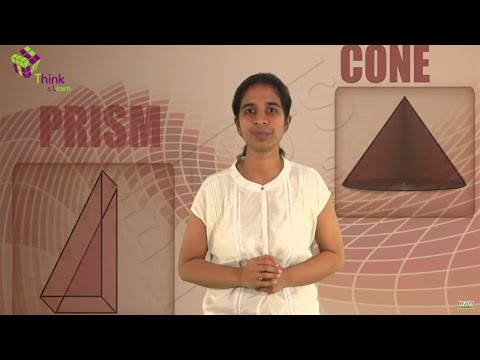### Nets of Solid Shapes

#### To Know About Nets Of Solid Shapes, Watch The Below Video: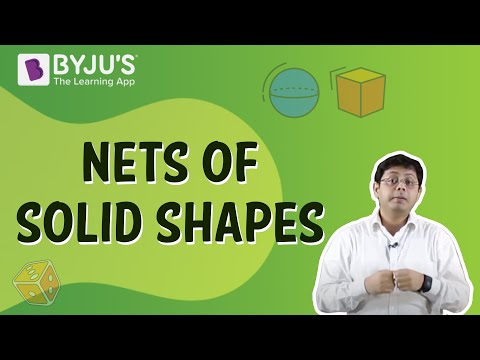### Three Dimensional Shapes Examples (Solved problems)

Example 1:

Find the volume of a cube if its side length is 6 cm.

Solution:

Given: Side length, a = 6 cm.

We know that the volume of cube = a3  cubic units.

Hence, V = 63 = 216 cm3

Hence, the volume of a cube is 216 cm3.

Example 2:

Find the total surface area of a sphere, whose radius is 3 cm. Use (π = 3.14)

Solution:

Given: Radius, r = 3 cm.

The formula to calculate the total surface area of a sphere is given by:

TSA of Sphere = 4πr² Square units

TSA of sphere = 4(3.14)(3)2 cm2

TSA of Sphere = 113.04 cm2.

Hence, the total surface area of a sphere is 113.04 cm2.

Example 3:

Find the volume of a cuboid, whose dimensions is 4cm × 6 cm × 12 cm.

Solution:

Given cuboid dimensions = 4cm × 6 cm × 12 cm

We know that the volume of a cuboid is lbh cubic units.

Hence, Volume of cuboid  = (4)(6)(12)  cm3.

V = 288 cm3

Therefore, the volume of the cuboid is 288 cm3.

### Practice Question on 3D Shapes Models in Maths

Solve the following problems on 3D shapes:

1. Find the volume of a cylinder whose radius is 4 cm and height is 8 cm.
2. Find the volume of a cone whose radius is 5 cm and height is 9 cm.
3. Find the volume of a pyramid whose base area is 126 cm2 and height is 10 cm.

## Frequently Asked Questions on Three Dimensional Shapes

### What are the three dimensional shapes?

The three-dimensional shape in geometry are those shapes that are defined along three dimensions such as length, width and height.

### What are the different types of three dimensional shapes?

The different types of three dimensional shapes are cone, cylinder, cuboid, cube, sphere, rectangular prism, pyramid.

### Is square a three dimensional shape?

Square is not a three dimensional shape, instead it is a two dimensional shape.

### What is a three dimensional round shape object called?

A three dimensional round shape is a sphere. For example, a football is a spherical shaped object.

### What are the examples of three dimensional shapes?

There are a number of examples of three dimensional shapes that can be seen in real life such as Rubik cube, a globe, gas cylinder, a cubical box, a cuboidal board etc.

Test your knowledge on Three Dimensional Shapes

#### 1 Comment

1. Ira Mistry

This was amazing but if you add some questions in it then it will be more easier